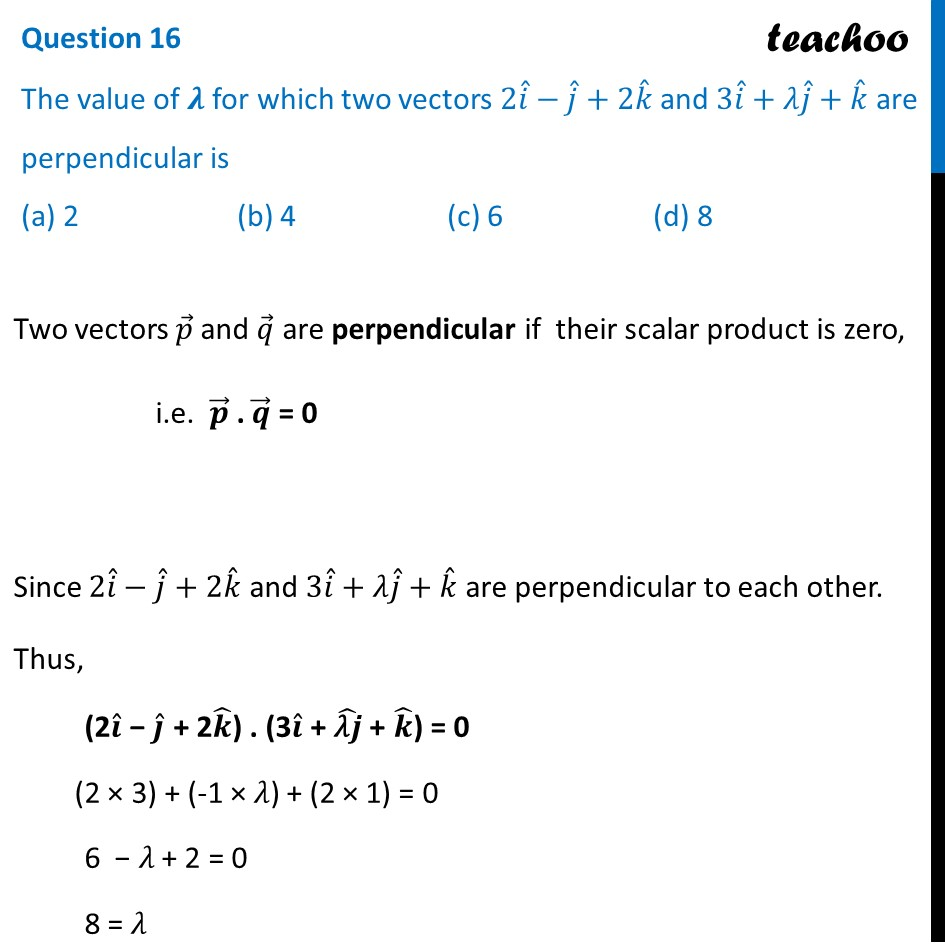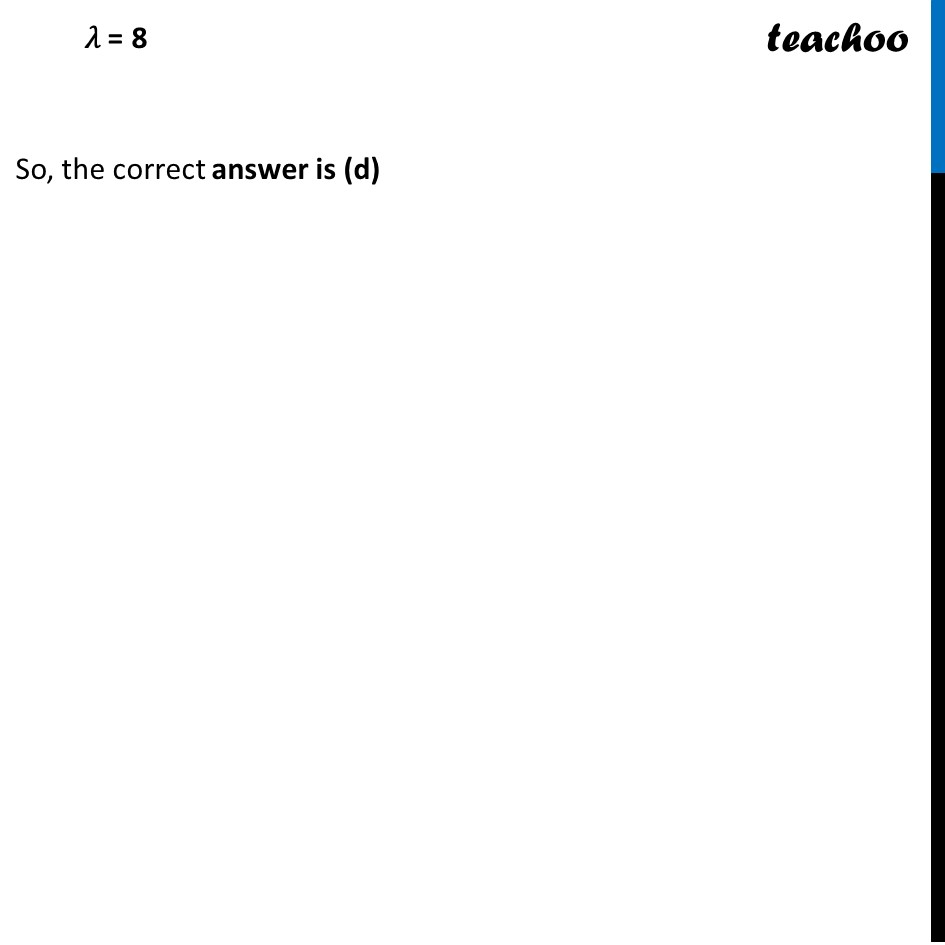CBSE Class 12 Sample Paper for 2024 Boards

Class 12
Solutions of Sample Papers and Past Year Papers - for Class 12 Boards

## (a) 2                      (b) 4                     (c) 6                     (d) 8

This question is similar to Question-23-Choice-1 CBSE Class 12 Sample Paper for 2023 BoardsLearn in your speed, with individual attention - Teachoo Maths 1-on-1 Class

### Transcript

Two vectors 𝑝 ⃗ and 𝑞 ⃗ are perpendicular if their scalar product is zero, i.e. 𝒑 ⃗ . 𝒒 ⃗ = 0 Since 2𝑖 ˆ−𝑗 ˆ+2𝑘 ˆ and 3𝑖 ˆ+𝜆𝑗 ˆ+𝑘 ˆ are perpendicular to each other. Thus, (2𝒊 ̂ − 𝒋 ̂ + 2𝒌 ̂) . (3𝒊 ̂ + ("𝜆" 𝒋) ̂ + 𝒌 ̂) = 0 (2 × 3) + (-1 × 𝜆) + (2 × 1) = 0 6 − 𝜆 + 2 = 0 8 = 𝜆 𝜆 = 8 So, the correct answer is (d)